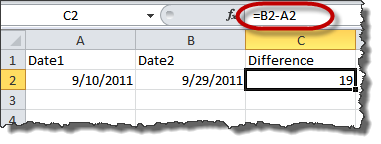# Reader Question: Calculate difference between two dates in Microsoft Excel

A reader contacted me recently with a deceptively simple Microsoft Excel question: “How do I calculate the difference between two dates?”

I say “deceptively simple” because the answer depends upon the context, namely, whether the two dates being compared are actually embedded in cells within the Microsoft Excel spreadsheet.

If they are, then the formula needed to perform the calculation is actually very simple (assuming you already know how to do simple formulas in Microsoft Excel):If, however, the dates are not anywhere else in the spreadsheet, you’ll have to embed them in the formula itself. This is done using the DATEVALUE function:What a lot of people don’t realize is that Microsoft Excel doesn’t actually store dates per se. It stores each date as a number calculated as the number of days from 1/1/1900, so that January 1, 1900 is “1” and all subsequent dates are successively higher numbers. The DATEVALUE function instructs Excel to convert the date back to its original number form in order to perform the arithmetic operation, in this case calculating the number of days between the two dates.

So the next time you find yourself needing to calculate the difference between the two dates, use a formula like one of those illustrated above.

I spend an inordinate amount of my time playing with computers and attempting to explain technology to lawyers and law office staff. It's not always easy, but someone's got to do it.

1.Blair McCune says:

This is a great topic. Obviously, correctly calculating time periods is critical for law offices. I sometimes need months and years elapsed, too. So, I use this function:

=DATEDIF([Start Date],[End Date],”[D/M/Y]”)

“D” gives you the days elapsed. “M” and “Y” give you the months and years elapsed.

I have a spreadsheet called AAACalculate that I use for this (and other) calculations. I label my A4 cell “Start Date” and my B4 cell “End Date.” The first function, =DATEDIF(A4,B4,”D”), goes in my C4 cell, which is labeled “Days Elapsed.” The second function, =DATEDIF(A4,B4,”M”), goes in my D4 cell, which is labeled “Months Elapsed.” The E4 cell has =DATEDIF(A4,B4,”Y”) for years elapsed.

Pull up the spreadsheet, type in the start and end dates, and you have the days, months, and years elapsed between the two dates.

1.Blair McCune says:

Sorry to reply to my own comment. I wanted to let you know that I found this function a long time ago. The function still seems to work OK, but it is not a “documented” function for newer versions of MS Excel.

2.The Guru says:

Cool technique – thanks for the tip, Blair!